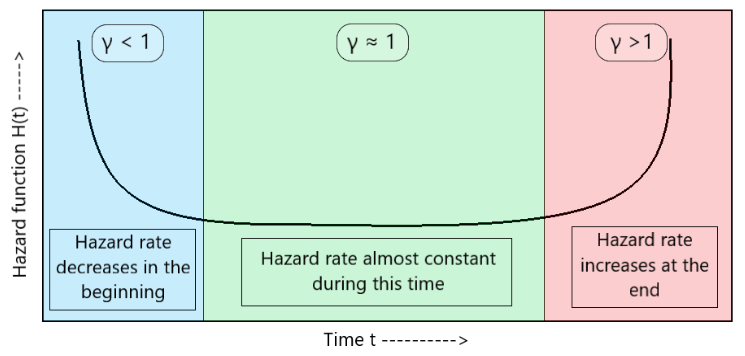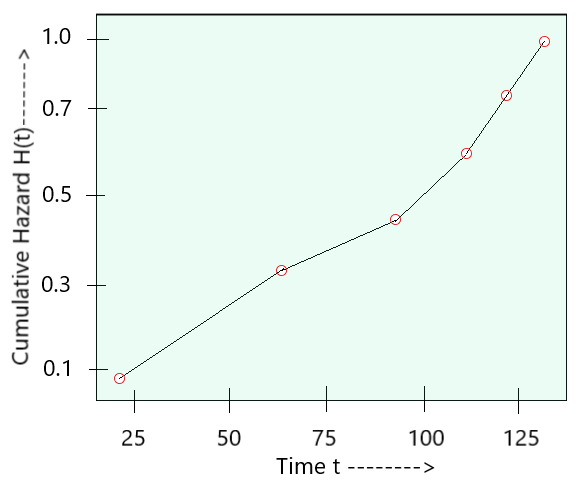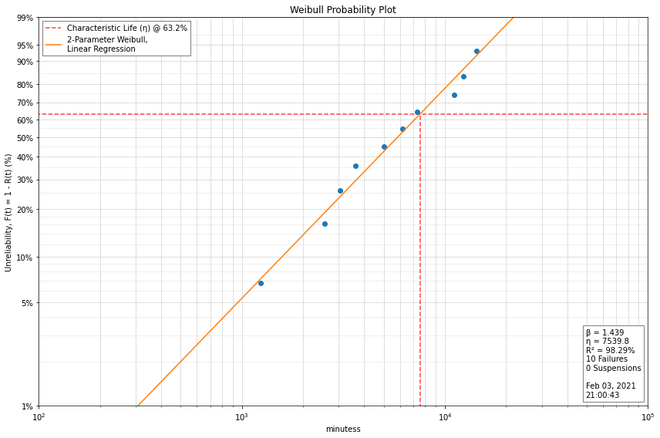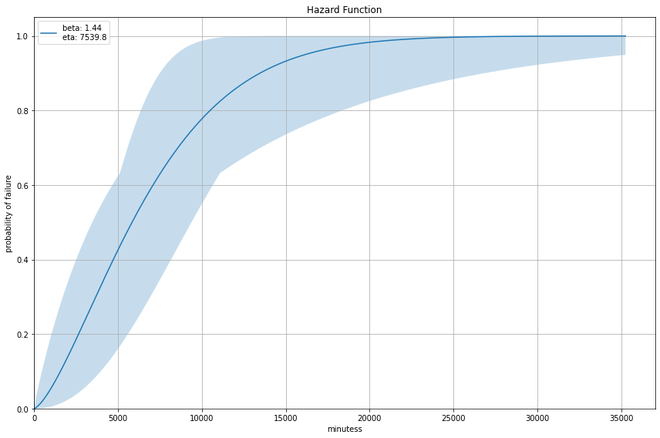Related Articles

# Weibull Hazard Plot

• Last Updated : 16 Mar, 2021

Before getting into the Weibull Hazard plot, let us first understand what a hazard function is.

Hazard function (h(t)): It tells us about the measure of risk of failure. It gives us information on the probability of failure of the object in a study in (t+1) time, assuming it has survived till time t. So the higher the hazard value, the higher is the risk of failure of the object in the study. A Hazard function for any object is represented as:

Cumulative Hazard Plot: It is a graphical representation that provides information on the reliability of the fitted model on the given dataset. (i.e. by looking at this plot, we can easily find the appropriate time of failure for a model. The cumulative hazard function for Weibull distribution is given by:where,
H(t) -> failure rate
t -> failure at time t
γ -> shape parameter
α -> scale parameter

Weibull Hazard function

The weibull distribution also has a hazard function h(t), that essentially tells us prior information about an event that is yet to take place. Let’s take an example for better understanding. Let us take an example for better understanding.

Let’s say we have a torch that we have been using for time t. The probability that it will fail at some time between t and t + dt hours of operation is given by the weibull hazard function. Based on the Weibull hazard plot, the hazard function for the torch can be described as:Just as in Weibull PPCC plot, hazard function h(t) also varies with the change in the shape parameter (γ) of the distribution. (See Fig 1)Fig 1: H(t) vs t plot

This plot works really well for 2-parameter weibull distributions that haven been discussed in the ‘Weibull Plot’ article.

Structure

The weibull hazard plot has the following parameters on the axes: (See Fig 2)

y-axis: Logarithm of hazard function, ln(H(t)).
x-axis: Logarithm of time, ln(t/α).

γ serves as the slope of this linear graph.Fig 2: Structure of Weibull Hazard plot

Manually Plotting a Weibull Hazard Plot

We know, that the Weibull hazard function isBy calculating the logarithmic values and plotting ln(H(t)) vs ln(t) plot, we get a linear-like plot. Hence we can determine a good estimate for the value of the shape parameter γ as it becomes the slope of that plot.

Code Implementation

Here is the code implementation showing a weibull plot and a corresponding weibull hazard plot.

 import weibull as wb# Creating a custom random data for failure points.fails = [5002.7, 6203.4, 14367.2, 11144.6, 7332.0,         3044.4, 12330.2, 1234.5, 2553.9, 3632.1]  # analysis contains the data to be fit into the weibull plot.Weibull_Analysis = wb.Analysis(fails, unit='minutes')  # fit() method used for fitting # the data into weibull plot.Weibull_Analysis.fit()  # printing the values of beta and eta (instances of Weibull_Analysis)print(f'beta: {Weibull_Analysis.beta: .03f}')print(f'eta: {Weibull_Analysis.eta: .03f}')  # probplot() method used # for plotting the weibull distributionWeibull_Analysis.probplot()  # hazard() method used for plotting# Weibull Hazard Plotprint(Weibull_Analysis.hazard())

OutputOutput 1 : Weibull PlotOutput 2 : Weibull Hazard Plot

Attention reader! Don’t stop learning now. Get hold of all the important Machine Learning Concepts with the Machine Learning Foundation Course at a student-friendly price and become industry ready.

My Personal Notes arrow_drop_up